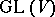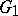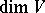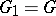# Lie-Kolchin theorem

(Redirected from Lie–Kolchin theorem)
Jump to: navigation, search

A solvable subgroupof the group(whereis a finite-dimensional vector space over an algebraically closed field) has a normal subgroupof index at most, wheredepends only on, such that inthere is a flagthat is invariant with respect to. In other words, there is a basis inin which the elements ofare written as triangular matrices. Ifis a connected closed subgroup ofin the Zariski topology, then; in this case the Lie–Kolchin theorem is a generalization of Lie's theorem, which was proved by S. Lie for complex connected (in the Euclidean topology) solvable Lie groups (see Lie group, solvable; Lie theorem). This assertion can also be considered as a special case of Borel's fixed-point theorem (cf. Borel fixed-point theorem).

The following analogue of the Lie–Kolchin theorem is true for an arbitrary field: A solvable group of matrices contains a normal subgroup of finite index whose commutator subgroup is nilpotent.

The Lie–Kolchin theorem was proved by E.R. Kolchin  (for connected groups) and A.I. Mal'tsev  (in the general formulation). It is also sometimes called the Kolchin–Mal'tsev theorem.